Chapter 5 : NMR proton: principles

A full section can be (and will be) dedicated to the detailed setup of the nucleus magnetic resonance (NMR) and to the analyses we can perform with this tool. In this section, we don’t need a lot of explanations on the NMR theory as it is a technique oriented section. We will however give an introduction on the NMR applied to the proton but keep in mind that a lot more can be done.

Principle of the NMR

Similarly to the spectroscopy, when a magnetic field is applied on a sample, some of the electromagnetic radiations can be absorbed, the frequencies of which depend on the properties of the sample. A NMR spectre give peaks of various intensities as a function of the frequency of absorption. Atoms have different reactions resulting from the application of the magnetic field and will absorb in separate regions of frequencies or won’t absorb at all. But more importantly, and that is what interests us in this section, the frequency of absorption of one atom depends on its direct environment. For instance, we can detect the difference of absorption for the hydrogen atoms at the extremity of the chain and in the chain in CH3-CH2-CH3 (δ≈0.91, 1.34, 0.91).

First, let’s come back to the absorption of different atoms. Any nucleus wears an electric charge but in some of them this charge turns in a given direction around the nuclear axis. This movement induces a magnetic dipole than can be described in term of number of nuclear spin I. I can have any positive value starting from 0 and by step of ½, i.e. 0, ½, 1, 3/2, 2, … One isotope has one value of spin that depends on the atomic mass and number of the isotope. We can resume this way: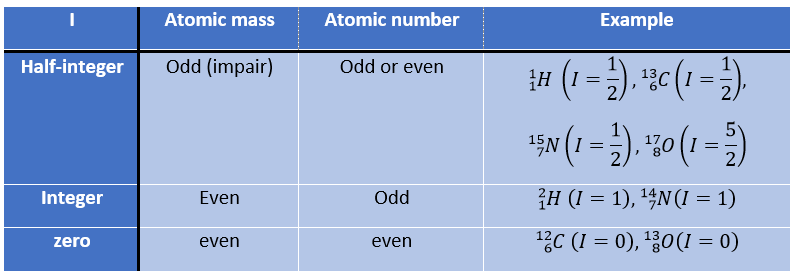The number of spin determines the number of orientations that a nucleus can take in a uniform external magnetic field, following the formula n=2I+1. An atom with I=0, such as the carbon 12, can thus only take one orientation and is “inactive” in NMR. Atoms with I≥1 have a non-spherical repartition of the charge described by a quadrupole moment that affects the nearby nuclei.

Atoms with I=1/2, such as 1H and 13C can take 2 orientations with different energies corresponding to the spin +1/2 and -1/2. The level of lower energy has a small excess of population that depends on the intensity of the applied magnetic field.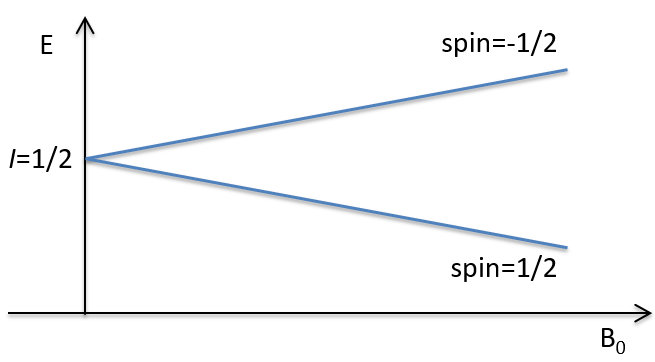To make the transition between the two levels, quanta’s of energy hν, with ν the frequency of the electromagnetic radiation, are needed in a magnetic field B0. The frequency is related to the magnetic field by the relation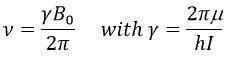γ is the gyromagnetic constant that depends on the spin I and on the magnetic moment μ. Knowing that, the problem is to find a way to transfer this energy and to measure the absorbed energy.

In a magnetic field, a proton turns on itself and around the axis of the magnetic field (see the next figure).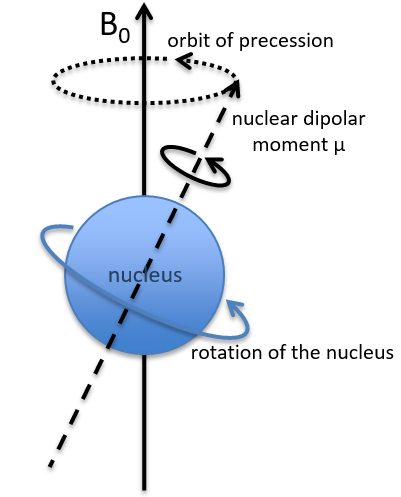The speed of precession ω0, also called frequency of Larmor is equal to the product of the gyromagnetic constant γ with the intensity of the magnetic field B0.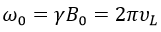Because of the magnetic field, all the atoms are oriented in the same direction but they are not at the same stage of the precession. Depending on the spin, the orientation in parallel to the field (spin=1/2) or antiparallel (spin=-1/2). As there is a small excess of population for the spin=1/2, a global magnetisation M0 is in the axis of B0 (axis z) but there is no particular magnetisation in the other directions (axes x-y).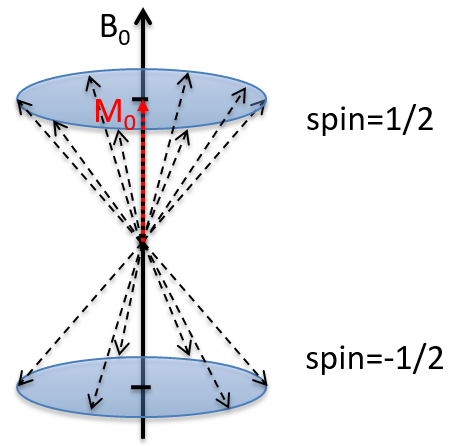We will tilt the magnetisation towards the horizontal plane using an oscillator that generates a second magnetic field B1 perpendicular to B0. The resulting electromagnetic field rotates at the same speed that the proton. We can detect the generated magnetic component in this plane (My for instance) if desired. Now that the nucleus is excited, we turn the magnetic field B1 off and let the nucleus relax back to its previous magnetisation.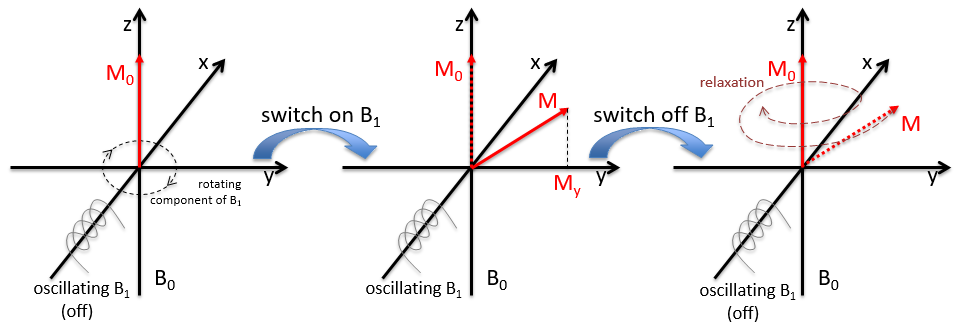Two methods of relaxation exist. The first is called spin-lattice relaxation or longitudinal relaxation and implies the transfer of the energy of the nuclei to the nearby molecules. This relaxation takes a times T1. The second mechanism of relaxation is the spin-spin relaxation, or transverse relaxation and implies the transfer of energy from one nucleus to a second one. It has a characteristic time T2. We can define R1 and R2 as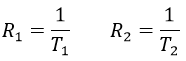To obtain a spectre of NMR, we scan either the frequency of the oscillator B1 or of the magnetic field B0.